## 请选出正确答案，共10题，每题10分，满分100分：

1. What is the largest decimal value that can be represented in binary using two bytes?

65,535

65,536

65,537

65,538

65,539

2. What is the result of (-67)-13 in binary, typically, two’s complement representation using a byte?

11001110

10011011

11000011

10110000

10001000

3. Write the expression for the output of figure below: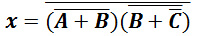4. Determine the magnitude of the resultant force acting on the screw eye and its direction measured clockwise from the x axis.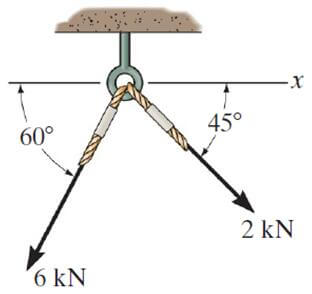6.20KN, 111.60°

6.40KN，103.40°

6.80KN, 103.40°

7.00KN，111.6°

7.20KN, 121.6°

5. Determine the tension in cables BA and BC necessary to support the 60kg cylinder in the follow diagram. (g=9.8N/kg)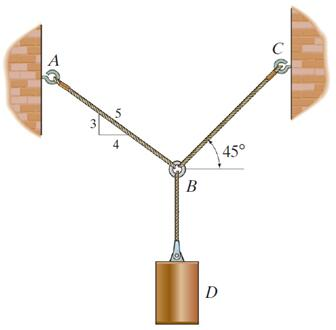$T_A=470N; T_B=410N$

$T_A=420N; T_B=476N$

$T_A=475N; T_B=415N$

$T_A=410N; T_B=466N$

$T_A=416N; T_B=426N$

6. Determine the moment of the force in the following diagram about point O.14.5 KN∙m; Anticlockwise

14.5 KN∙m; Clockwise

15.4 KN∙m; Clockwise

15.4 KN∙m; Anticlockwise

15.4 KN∙m; No direction

7. A man of mass 70kg and a boy of mass 35kg are standing together on a smooth ice surface. They push each other apart so that the man moves away with a speed of 0.30m/s. What is the final speed of the boy?

0.55 m/s

0.58m/s

0.60m/s

0.65m/s

0.69m/s

8. For the circuit element, which one of the following statements is true?

Voltage source is passive element.

Inductor is active element.

Current source is passive element.

Capacitor is active element.

Resistor is passive element.

9. Find the equivalent resistance in the given circuit.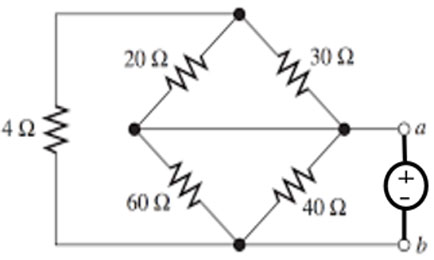$R_{eq}=10.3Ω$

$R_{eq}=9.4Ω$

$R_{eq}=9.8Ω$

$R_{eq}=9.6Ω$

$R_{eq}=9.2Ω$

10. Find the voltage V in the following diagram.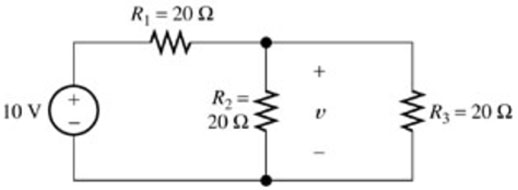2.22V

3.33V

3.44V

4.33V

5.33V• 获取验证码